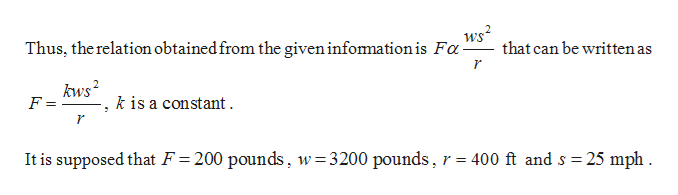# The force needed to keep a car from skidding on a curve varies inversely as the radius of the curve and jointly as the weight of the car and the square of the car's speed. Suppose that 200pounds of force keeps a 3200-pound car from skidding on a curve of radius 400ftat 25mph. What force would keep the same car from skidding on a curve of radius 550ftat 55mph?

Question
327 views

The force needed to keep a car from skidding on a curve varies inversely as the radius of the curve and jointly as the weight of the car and the square of the car's speed. Suppose that
200

pounds of force keeps a
3200

-pound car from skidding on a curve of radius
400
ft

at
25
mph

. What force would keep the same car from skidding on a curve of radius
550
ft

at
55
mph

?

check_circle

Step 1

Given: The Force (F) that is needed to keep a car from skidding on a curve varies inversely as the radius (r) of the curve, and jointly as the weight (w) of the car and square of the car’s speed (s).help_outlineImage Transcriptionclosews2 that can be written as Thus, the relation obtained from the given infomation is Fa kws F = k is a constant r 400 ft and s 25 mph It is supposed that F = 200 pounds, w=3200 pounds fullscreen
Step 2

In order to obtain the force that would keep the same car on a radius of 550 ft at 55 mph, assume w as constant.

Step 3

Obtain the value of ...

### Want to see the full answer?

See Solution

#### Want to see this answer and more?

Solutions are written by subject experts who are available 24/7. Questions are typically answered within 1 hour.*

See Solution
*Response times may vary by subject and question.
Tagged in

### Algebra